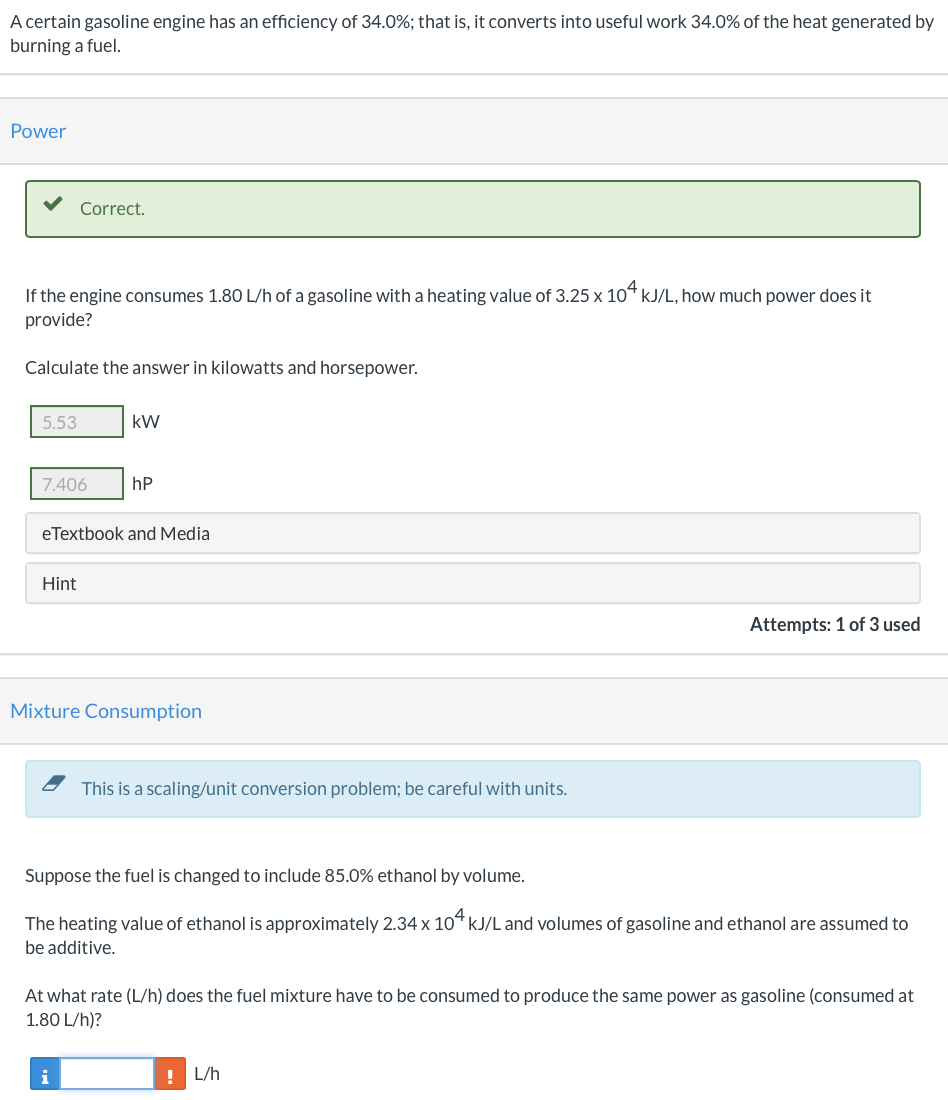# Question Solved1 AnswerNeed help with the last question! A certain gasoline engine has an efficiency of 34.0%; that is, it converts into useful work 34.0% of the heat generated by burning a fuel. Power Correct. If the engine consumes 1.80 L/h of a gasoline with a heating value of 3.25 x 104 kJ/L, how much power does it provide? Calculate the answer in kilowatts and horsepower. 5.53 kW 7.406 hp e Textbook and Media Hint Attempts: 1 of 3 used Mixture Consumption This is a scaling/unit conversion problem; be careful with units. Suppose the fuel is changed to include 85.0% ethanol by volume. The heating value of ethanol is approximately 2.34 x 104 kJ/L and volumes of gasoline and ethanol are assumed to be additive. At what rate (L/h) does the fuel mixture have to be consumed to produce the same power as gasoline (consumed at 1.80 L/h)? i ! L/hNeed help with the last question!Transcribed Image Text: A certain gasoline engine has an efficiency of 34.0%; that is, it converts into useful work 34.0% of the heat generated by burning a fuel. Power Correct. If the engine consumes 1.80 L/h of a gasoline with a heating value of 3.25 x 104 kJ/L, how much power does it provide? Calculate the answer in kilowatts and horsepower. 5.53 kW 7.406 hp e Textbook and Media Hint Attempts: 1 of 3 used Mixture Consumption This is a scaling/unit conversion problem; be careful with units. Suppose the fuel is changed to include 85.0% ethanol by volume. The heating value of ethanol is approximately 2.34 x 104 kJ/L and volumes of gasoline and ethanol are assumed to be additive. At what rate (L/h) does the fuel mixture have to be consumed to produce the same power as gasoline (consumed at 1.80 L/h)? i ! L/h
More
Transcribed Image Text: A certain gasoline engine has an efficiency of 34.0%; that is, it converts into useful work 34.0% of the heat generated by burning a fuel. Power Correct. If the engine consumes 1.80 L/h of a gasoline with a heating value of 3.25 x 104 kJ/L, how much power does it provide? Calculate the answer in kilowatts and horsepower. 5.53 kW 7.406 hp e Textbook and Media Hint Attempts: 1 of 3 used Mixture Consumption This is a scaling/unit conversion problem; be careful with units. Suppose the fuel is changed to include 85.0% ethanol by volume. The heating value of ethanol is approximately 2.34 x 104 kJ/L and volumes of gasoline and ethanol are assumed to be additive. At what rate (L/h) does the fuel mixture have to be consumed to produce the same power as gasoline (consumed at 1.80 L/h)? i ! L/h